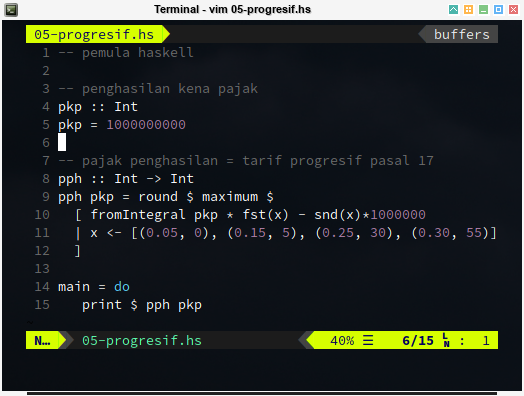# Haskell - Tax Tariff - Part Three

Table of Content

### Alternative Calculation

Just like what I said in previous, we can rid of the guard.

#### Using List and Maximum

List come to the rescue.

``````-- taxable incomes
pkps :: [Int]
pkps = [50*10^6, 250*10^6, 500*10^6, 10^9]

-- income tax using progressive tariff
pph :: Int -> Int
pph pkp = maximum \$ [round(x) | x <- pph_list]
where
-- Typecast, such as to Double
pkp_d = fromIntegral(pkp)
-- to avoid typo in writing digit
million = 10^6
-- tariff rate in percent
pph_p = [0.05, 0.15, 0.25, 0.30]
-- difference, short version
pph_d = [0, 5, 30, 55]
-- difference, in million rupiah
pph_dj = map (fromIntegral <\$> (*million)) pph_d
-- range list, zero based index
level   = [0, 1, 2, 3]
-- main calculation, calulate all level
pph_list = [pkp_d * (pph_p !! x) - (pph_dj !! x) | x <- level]

main = print \$ map pph pkps``````

Code above will have the result as below

``````2500000
32500000
95000000
245000000``````

The downside of the method in code above is, all four ranges calculated first before finding the `maximum` value. It does not looks efficient.

Can the code be shorter ?

#### Dictionary

We can compress the list further into dictionary.

``````-- [(percentage, difference)]
tariffSchema :: [(Double, Int)]
tariffSchema =
[(0.05,  0)
,(0.15,  5)
,(0.25, 30)
,(0.30, 55)
]``````

#### First and Second

And access the value using, `fst` (first) and `snd` (second) as below code

``````  [ fromIntegral pkp * fst(x) - snd(x) * (1000000)
| x <- [(0.05, 0), (0.15, 5), (0.25, 30), (0.30, 55)]
]``````

#### Simply List

Or even simpler, just a list.

``````-- taxable incomes
pkps :: [Int]
pkps = [50*10^6, 250*10^6, 500*10^6, 10^9]

-- income tax using progressive tariff
pph :: Int -> Int
pph pkp = round \$ maximum \$
[ fromIntegral pkp * a - b * (10^6)
| (a, b) <- [(0.05, 0), (0.15, 5), (0.25, 30), (0.30, 55)]
]

main = mapM_ (print . pph) pkps``````

Code above will also result some lines for as below

``````2500000
32500000
95000000
245000000``````The final code doesn’t feel as clear as our first post. And also it is not efficient as it has to calculate all four ranges.

### List Comprehensions

The issue with `maximum` can be solved. With `last` I found the right range, and calculate only what I need.

``````import Data.Foldable

-- taxable incomes
pkps :: [Int]
pkps = [50*10^6, 250*10^6, 500*10^6, 10^9]

-- income tax using progressive tariff
pph :: Int -> Int
pph pkp = round \$
(\(t, p, d) -> fromIntegral pkp * p - d * (10^6))
(reff !! last indices)
where
-- [(level, percentage, diff)]
reff    = [(0, 0.05, 0), (50, 0.15, 5), (250, 0.25, 30), (500, 0.30, 55)]
indices = [ i | (i, (t, p, d)) <- zip [0..] reff, pkp >= (t * 10^6) ]

main = forM_ pkps (print . pph)``````

Notice that I also import the `Data.Foldable`, so that we can have for loop like syntax.

``````import Data.Foldable

...

main = forM_ pkps (print . pph)``````

Code above will display the result line by line for each calculatin, as shown in below output:

``````2500000
32500000
95000000
245000000``````

This code looks so cryptic. And it also longer than the previous one.

I guess I fail miserably.

### What is Next ?

What do you think, the coolest code, between this four article?

We can go back to original calculation code from the first article, and let’s see if we can make simplified the code. Consider continue reading [ Haskell - Tax Tariff - Part Four ].

Thank you for visiting.